# Cyclic group

In algebra, a cyclic group or monogenous group is a group that is generated by a single element. That is, it consists of a set of elements with a single invertible associative operation, and it contains an element g such that every other element of the group may be obtained by repeatedly applying the group operation or its inverse to g. Each element can be written as a power of g in multiplicative notation, or as a multiple of g in additive notation. This element g is called a generator of the group.

Every infinite cyclic group is isomorphic to the additive group of Z, the integers. Every finite cyclic group of order n is isomorphic to the additive group of Z/nZ, the integers modulo n. Every cyclic group is an abelian group (meaning that its group operation is commutative), and every finitely generated abelian group is a direct product of cyclic groups.

## Definition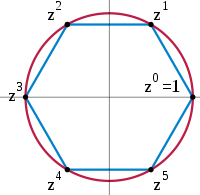The six 6th complex roots of unity form a cyclic group under multiplication. z is a primitive element, but z2 is not, because the odd powers of z are not a power of z2.
Infinite cyclic groups
p1, (*∞∞) p11g, (22∞)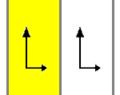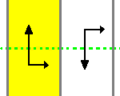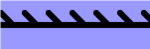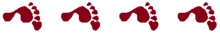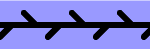Two frieze groups are isomorphic to Z. With one generator, p1 has translations and p11g has glide reflections.

A group G is called cyclic if there exists an element g in G such that G = ⟨g⟩ = { gn | n is an integer }. Since any group generated by an element in a group is a subgroup of that group, showing that the only subgroup of a group G that contains g is G itself suffices to show that G is cyclic.

For example, if G = { g0, g1, g2, g3, g4, g5 } is a group of order 6, then g6 = g0, and G is cyclic. In fact, G is essentially the same as (that is, isomorphic to) the set { 0, 1, 2, 3, 4, 5 } with addition modulo 6. For example, 1 + 2 ≡ 3 (mod 6) corresponds to g1 · g2 = g3, and 2 + 5 ≡ 1 (mod 6) corresponds to g2 · g5 = g7 = g1, and so on. One can use the isomorphism χ defined by χ(gi) = i.

The name "cyclic" may be misleading: it is possible to generate infinitely many elements and not form any literal cycles; that is, every gn is distinct. (It can be thought of as having one infinitely long cycle.) A group generated in this way (for example, the first frieze group, p1) is called an infinite cyclic group, and is isomorphic to the additive group of the integers, (Z, +).

Nicolas Bourbaki, who was the French mathematicians group, called a cyclic group as monogenous group,[note 1] and defined a finite monogenous group as a cyclic group,[note 1] then, Bourbaki avoided the term infinite cyclic group.

Definition of a cyclic group
Finite case Infinite case
Finite cyclic group Infinite cyclic group
Bourbaki's definition Monogenous group
Finite monogenous group
(= cyclic group)
Infinite monogenous group

## Examples

C1 C2 C3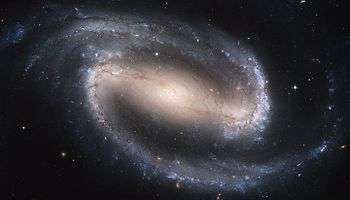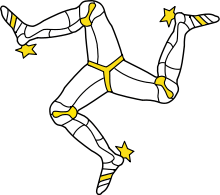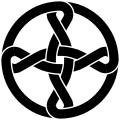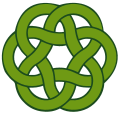The set of integers, with the operation of addition, forms a group. It is an infinite cyclic group, because all integers can be written as a finite sum or difference of copies of the number 1. In this group, 1 and −1 are the only generators. Every infinite cyclic group is isomorphic to this group.

For every positive integer n, the set of integers modulo n, again with the operation of addition, forms a finite cyclic group, the group Z/n. An element g is a generator of this group if g is relatively prime to n. Thus, the number of different generators is φ(n), where φ is the Euler totient function, the function that counts the number of numbers modulo n that are relatively prime to n. Every finite cyclic group is isomorphic to a group Z/n, where n is the order of the group.

The integer and modular addition operations, used to define the cyclic groups, are both the addition operations of commutative rings, also denoted Z and Z/n. If p is a prime, then Z/p is a finite field, and is usually instead written as Fp or GF(p). Every field with p elements is isomorphic to this one.

### Modular multiplication

For every positive integer n, the subset of the integers modulo n that are relatively prime to n, with the operation of multiplication, forms a finite group that for many values of n is again cyclic. It is the group under multiplication modulo n, and it is cyclic whenever n is 1, 2, 4, a power of an odd prime, or twice a power of an odd prime. (sequence A033948 in the OEIS)  Its elements are the units of the ring Z/nZ; there are φ(n) of them, where again φ is the totient function. This group is written as (Z/nZ)×. For example, (Z/6Z)× has as its elements {1,5}; 6 is twice a prime, so this is a cyclic group. In contrast, (Z/8Z)× (with elements {1,3,5,7}) is the Klein group and is not cyclic. When (Z/nZ)× is cyclic, every generator of (Z/nZ)× is called a primitive root modulo n.

The cyclic group (Z/pZ)× for a prime number p, is also written (Z/pZ)* because it consists of the non-zero elements of the finite field of order p. More generally, every finite subgroup of the multiplicative group of any field is cyclic.

### Rotational symmetries

Main article: Rotational symmetry

The set of rotational symmetries of a polygon forms a finite cyclic group. If there are n different ways of mapping the polygon to itself by a rotation (including the null rotation) then this group is isomorphic to Zn. In three or higher dimensions there can exist other finite symmetry groups that are cyclic, but that do not form the set of rotations around a single axis.

The group S1 of all rotations of a circle (the circle group) is not cyclic. Unlike the infinite cyclic group, it is not even countable. There also exist other infinite rotation groups (such as the set of rotations by rational angles) that are countable but not cyclic.

### Galois theory

An nth root of unity may be thought of as a complex number whose nth power is 1. That is, it is a root of the polynomial xn  1. The nth roots of unity form a cyclic group of order n under multiplication. For example, the polynomial 0 = z3 − 1 factors as (zs0)(zs1)(zs2), where s = ei/3; the set {s0, s1, s2} forms a cyclic group under multiplication. The Galois group of the field extension of the rational numbers generated by the nth roots of unity forms a different group. It is isomorphic to the multiplicative group modulo n, which has order φ(n) and is cyclic for some but not all n.

A field extension is called a cyclic extension if its Galois group is a cyclic group. The Galois group of every finite extension of a finite field is finite and cyclic, with an iterate of the Frobenius endomorphism as its generator. Conversely, given a finite field F and a finite cyclic group G, there is a finite field extension of F whose Galois group is G.

## Subgroups and notation

All subgroups and quotient groups of cyclic groups are cyclic. Specifically, all subgroups of Z are of the form mZ, with m an integer ≥0. All of these subgroups are distinct from each other, and apart from the trivial group (for m = 0) all are isomorphic to Z. The lattice of subgroups of Z is isomorphic to the dual of the lattice of natural numbers ordered by divisibility. In particular, because the prime numbers are the numbers with no nontrivial divisors, a cyclic group is simple if and only if its order (the number of its elements) is prime.

Since the cyclic groups are abelian, they are often written additively and denoted Zn with the identity written 0. However, this notation can be problematic for number theorists because it conflicts with the usual notation for p-adic number rings or localization at a prime ideal. The quotient notations Z/nZ, Z/n, and Z/(n) are standard alternatives.

One may instead write the group multiplicatively, and denote it by Cn, where n is the order for finite groups and by C for the infinite cyclic group.[note 2] For example, g2g4 = g1 in C5, whereas 2 + 4 = 1 in Z/5Z.

All quotient groups of Z are finite, except for the trivial exception Z/{0} = Z/0Z. For every positive divisor d of n, the quotient group Z/nZ has precisely one subgroup of order d, the one generated by the residue class of n/d. There are no other subgroups. Using the quotient group formalism, Z/nZ is a standard notation for the additive cyclic group with n elements. In ring terminology, the subgroup nZ is also the ideal (n), so the quotient can also be written Z/(n) or Z/n without abuse of notation. These alternatives do not conflict with the notation for the p-adic integers. The last form is very common in informal calculations; it has the additional advantage that it reads the same way that the group or ring is often described verbally in English, "Zee mod en".

Every cyclic group is abelian. That is, its group operation is commutative: gh = hg (for all g and h in G). This is clear for the groups of integer and modular addition since r + ss + r (mod n), and it follows for all cyclic groups since they are all isomorphic to a group generated by an addition operation. For a finite cyclic group of order n, and every element e of the group, en is the identity element of the group. This again follows by using the isomorphism to modular addition, since kn ≡ 0 (mod n) for every integer k.

If d is a divisor of n, then the number of elements in Z/n which have order d is φ(d), and the number of elements whose order divides d is exactly d. If G is a finite group in which, for each n > 0, G contains at most n elements of order dividing n, then G must be cyclic.[note 3] The order of an element m of the group is n/gcd(n,m).

The direct product of two cyclic groups Z/n and Z/m is cyclic if and only if n and m are coprime. Thus e.g. Z/12 is the direct product of Z/3 and Z/4, but not the direct product of Z/6 and Z/2. If p is a prime number, then the only group (up to isomorphism) with p elements is Z/p. it is called a primary cyclic group. The fundamental theorem of abelian groups states that every finitely generated abelian group is the direct product of finitely many finite primary cyclic and infinite cyclic groups. A number n is called a cyclic number if it has the property that Z/n is the only group of order n, which is true exactly when gcd(n,φ(n)) = 1. The cyclic numbers include all prime numbers, but also include some composite numbers such as 15. However, except 2, all cyclic numbers are odd. The cyclic numbers are:

1, 2, 3, 5, 7, 11, 13, 15, 17, 19, 23, 29, 31, 33, 35, 37, 41, 43, 47, 51, 53, 59, 61, 65, 67, 69, 71, 73, 77, 79, 83, 85, 87, 89, 91, 95, 97, 101, 103, 107, 109, 113, 115, 119, 123, 127, 131, 133, 137, 139, 141, 143, ... (sequence A003277 in the OEIS)

In fact, a number n is a cyclic number if and only if gcd(n, φ(n)) = 1, where φ is the Euler's totient function.

The definition immediately implies that cyclic groups have group presentation C = x | and Cn = x | xn for finite n.

## Associated objects

### Representations

The representation theory of the cyclic group is a critical base case for the representation theory of more general finite groups. In the complex case, a representation of a cyclic group decomposes into a direct sum of linear characters, making the connection between character theory and representation theory transparent. In the positive characteristic case, the indecomposable representations of the cyclic group form a model and inductive basis for the representation theory of groups with cyclic Sylow subgroups and more generally the representation theory of blocks of cyclic defect.

### Cycle graph

Further information: Cycle graph (algebra)

A cycle graph illustrates the various cycles of a group and is particularly useful in visualizing the structure of small finite groups. A cycle graph for a cyclic group is simply a circular graph, where the group order is equal to the number of nodes. A single generator defines the group as a directional path on the graph, and the inverse generator defines a backwards path. Trivial paths (identity) can be drawn as a loop but are usually suppressed. Z2 is sometimes drawn with two curved edges as a multigraph.

Cyclic groups Zn, order n, is a single cycle graphed simply as an n-sided polygon with the elements at the vertices. A cyclic group Zn can be decomposed into a direct product Za×Zb where n=ab, where a and b are relatively prime (gcd(a,b)=1).

 Z1 Z2 Z3 Z4 Z5 Z6=Z3×Z2 Z7 Z8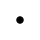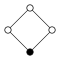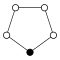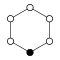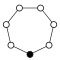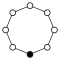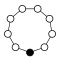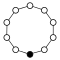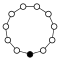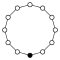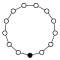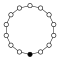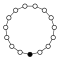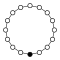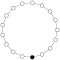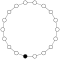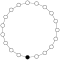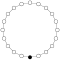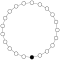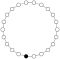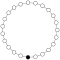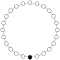### Cayley graph

Main article: Circulant graph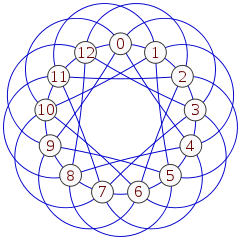The Paley graph of order 13, a circulant graph formed as the Cayley graph of Z/13 with generator set {1,3,4}

A Cayley graph is a graph defined from a pair (G,S) where G is a group and S is a set of generators for the group; it has a vertex for each group element, and an edge for each product of an element with a generator. In the case of a finite cyclic group, with its single generator, the Cayley graph is a cycle graph, and for an infinite cyclic group with its generator the Cayley graph is a doubly infinite path graph. However, Cayley graphs can be defined from other sets of generators as well. The Cayley graphs of cyclic groups with arbitrary generator sets are called circulant graphs. These graphs may be represented geometrically as a set of equally spaced points on a circle or on a line, with each point connected to neighbors with the same set of distances as each other point. They are exactly the vertex-transitive graphs whose symmetry group includes a transitive cyclic group.

### Endomorphisms

The endomorphism ring of the abelian group Z/nZ is isomorphic to Z/nZ itself as a ring. Under this isomorphism, the number r corresponds to the endomorphism of Z/nZ that maps each element to the sum of r copies of it. This is a bijection if and only if r is coprime with n, so the automorphism group of Z/nZ is isomorphic to the unit group (Z/nZ)×.

Similarly, the endomorphism ring of the additive group of Z is isomorphic to the ring Z. Its automorphism group is isomorphic to the group of units of the ring Z, i.e. to ({−1, +1}, ×) ≅ C2.

### Tensor product and Hom of cyclic groups

The tensor productand the group of homomorphismscan be shown to both be isomorphic to.

For the tensor product, this is a consequence of the general fact. For the Hom group, recall that it is isomorphic to the subgroup ofconsisting of the elements of order dividing m. That subgroup is cyclic of order gcd (m, n), which completes the proof.

## Related classes of groups

Several other classes of groups have been defined by their relation to the cyclic groups:

### Virtually cyclic groups

A group is called virtually cyclic if it contains a cyclic subgroup of finite index (the number of cosets that the subgroup has). In other words, any element in a virtually cyclic group can be arrived at by applying a member of the cyclic subgroup to a member in a certain finite set. Every cyclic group is virtually cyclic, as is every finite group. An infinite group is virtually cyclic if and only if it is finitely generated and has exactly two ends;[note 4] an example of such a group is the product of Z/n and Z, in which the Z factor has finite index n. Every abelian subgroup of a Gromov hyperbolic group is virtually cyclic.

### Locally cyclic groups

Main article: Locally cyclic group

A locally cyclic group is a group in which each finitely generated subgroup is cyclic. An example is the additive group of the rational numbers: every finite set of rational numbers is a set of integer multiples of a single unit fraction, the inverse of their lowest common denominator, and generates as a subgroup a cyclic group of integer multiples of this unit fraction. A group is locally cyclic if and only if its lattice of subgroups is a distributive lattice.

### Cyclically ordered groups

A cyclically ordered group is a group together with a cyclic order preserved by the group structure. Every cyclic group can be given a structure as a cyclically ordered group, consistent with the ordering of the integers (or the integers modulo the order of the group). Every finite subgroup of a cyclically ordered group is cyclic.

### Metacyclic and polycyclic groups

A metacyclic group is a group containing a cyclic normal subgroup whose quotient is also cyclic. These groups include the cyclic groups, the dicyclic groups, and the direct products of two cyclic groups. The polycyclic groups generalize metacyclic groups by allowing more than one level of group extension. A group is polycyclic if it has a finite descending sequence of subgroups, each of which is normal in the previous subgroup with a cyclic quotient, ending in the trivial group. Every finitely generated abelian group or nilpotent group is polycyclic.

## Footnotes

### Notes

1. DEFINITION 15. A group is called monogenous if it admits a system of generators consisting of a single element. A finite monogenous group is called cyclic.
Bourbaki, Algebra 1, Chapter 3, p.48
2. The infinite cyclic group written multiplicatively may be written C (most common), C (the subscript being interpreted as the order of the group), or even C0 (to fit the statement CnZ/nZ, n ≥ 0, as given cyclic group in nLab).
3. This implication remains true even if only prime values of n are considered. See Gallian (2010, p. 84, Exercise 43). (And observe that when n is prime, there is exactly one element whose order is a proper divisor of n, namely the identity.)
4. See Stallings (1970, pp. 124–128). See in particular p. 126: "If G has two ends, the explicit structure of G is well known: G is an extension of a finite group by either the infinite cyclic group or the infinite dihedral group."

### Citations

1. Hazewinkel, Michiel, ed. (2001), "Cyclic group", Encyclopedia of Mathematics, Springer, ISBN 978-1-55608-010-4
2. (Lajoie & Mura 2000, pp. 29–33).
3. (Bourbaki 1998, p. 48)
4. (Vinogradov 2003, pp. 105–132, § VI PRIMITIVE ROOTS AND INDICES).
5. (Motwani & Raghavan 1995, p. 401).
6. (Rotman 1998, p. 65).
7. (Stewart & Golubitsky 2010, pp. 47–48).
8. (Cox 2012, p. 294, Theorem 11.1.7).
9. (Cox 2012, p. 295, Corollary 11.1.8 and Theorem 11.1.9).
10. (Aluffi 2009, pp. 82–84, 6.4 Example: Subgroups of Cyclic Groups).
11. (Gannon 2006, p. 18).
12. (Jungnickel 1992, pp. 545–547).
13. (Coxeter & Moser 1980, p. 1).
14. (Alspach 1997, pp. 1–22).
15. (Vilfred 2004, pp. 34–36).
16. (Kurzweil & Stellmacher 2004, p. 50).
17. (Alonso 1991, Corollary 3.6).
18. (Ore 1938, pp. 247–269).
19. (Fuchs 2011, p. 63).
20. A. L. Shmel'kin (2001), "Metacyclic group", in Hazewinkel, Michiel, Encyclopedia of Mathematics, Springer, ISBN 978-1-55608-010-4
21. Hazewinkel, Michiel, ed. (2001), "Polycyclic group", Encyclopedia of Mathematics, Springer, ISBN 978-1-55608-010-4